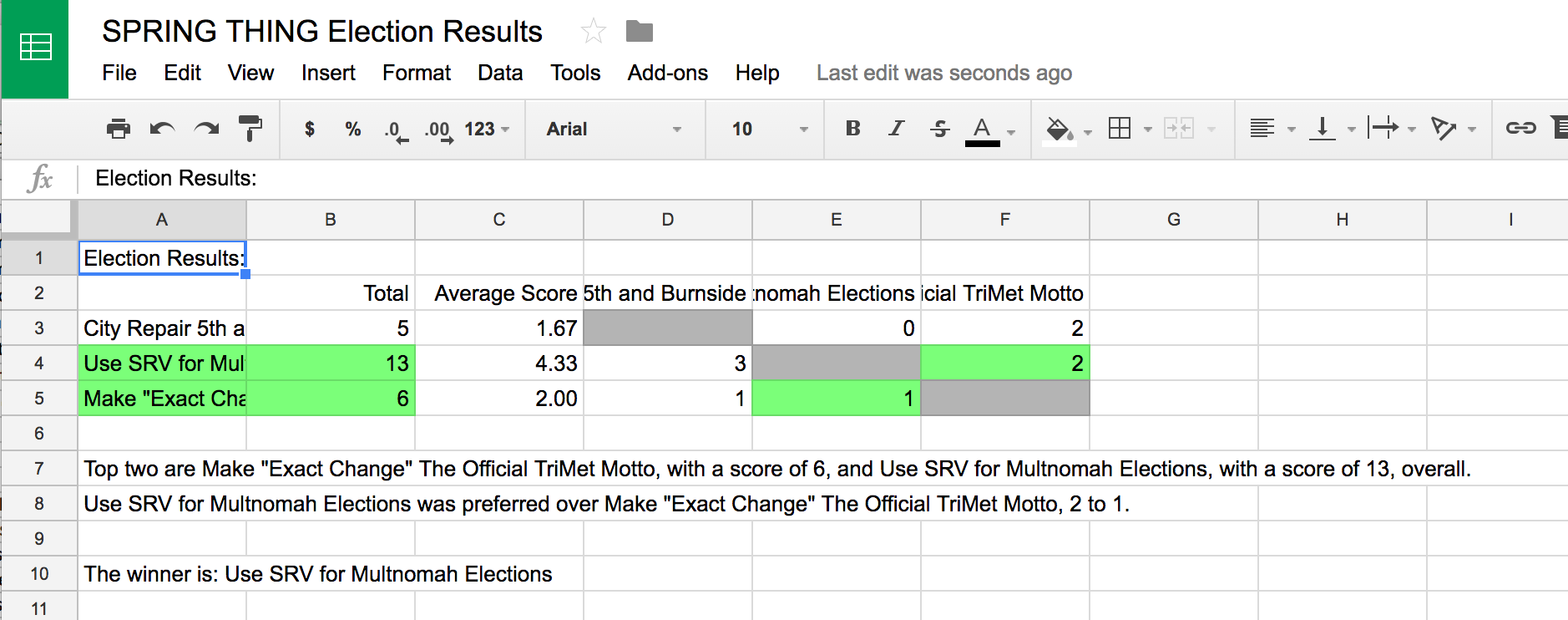## Star Voting (SRV) Election Calculator

With Google Forms and a simple script, it's easy to run your own Star Voting elections. This post is a step-by-step guide that shows how.

Step 1: Create a new Google Form for your election

Go to Google Forms and create a new form.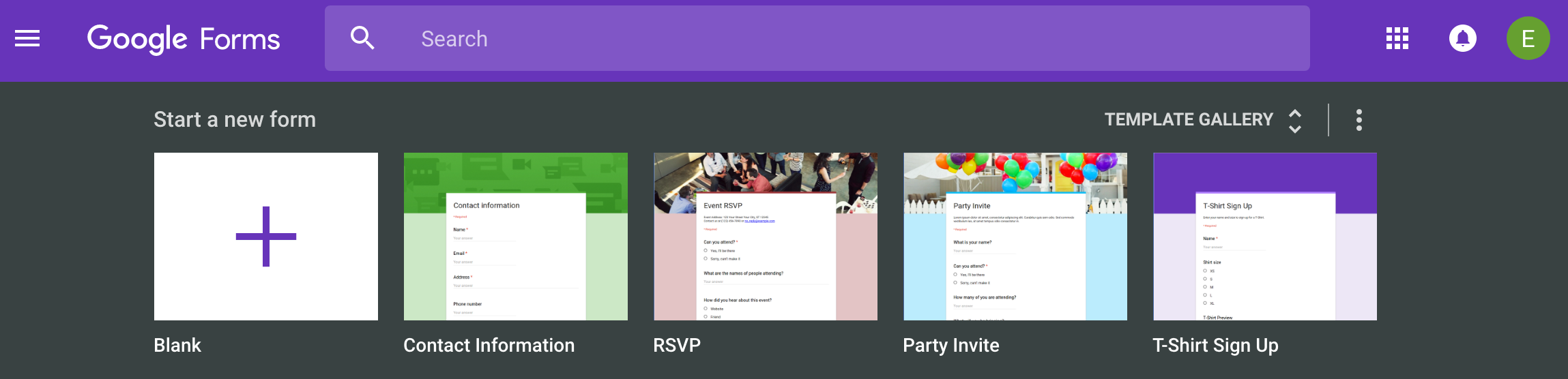Step 2: Name your election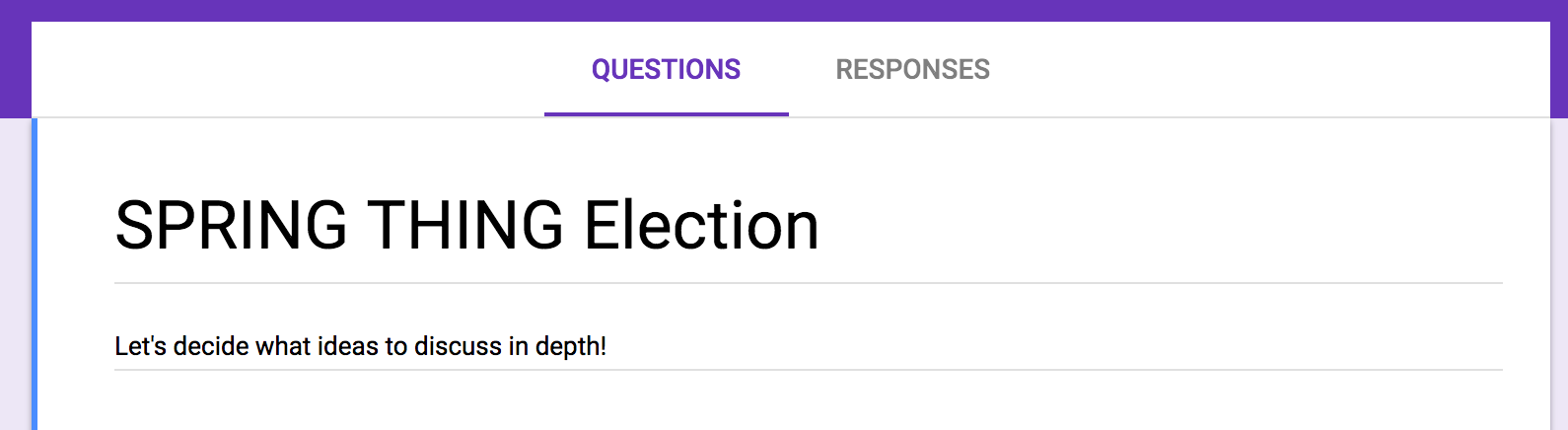Step 3: Choose the "Linear Scale" item type for the first entry

Each entry on the ballot should use the "Linear Scale" item type. This gives the voters a set of radio buttons for the score options.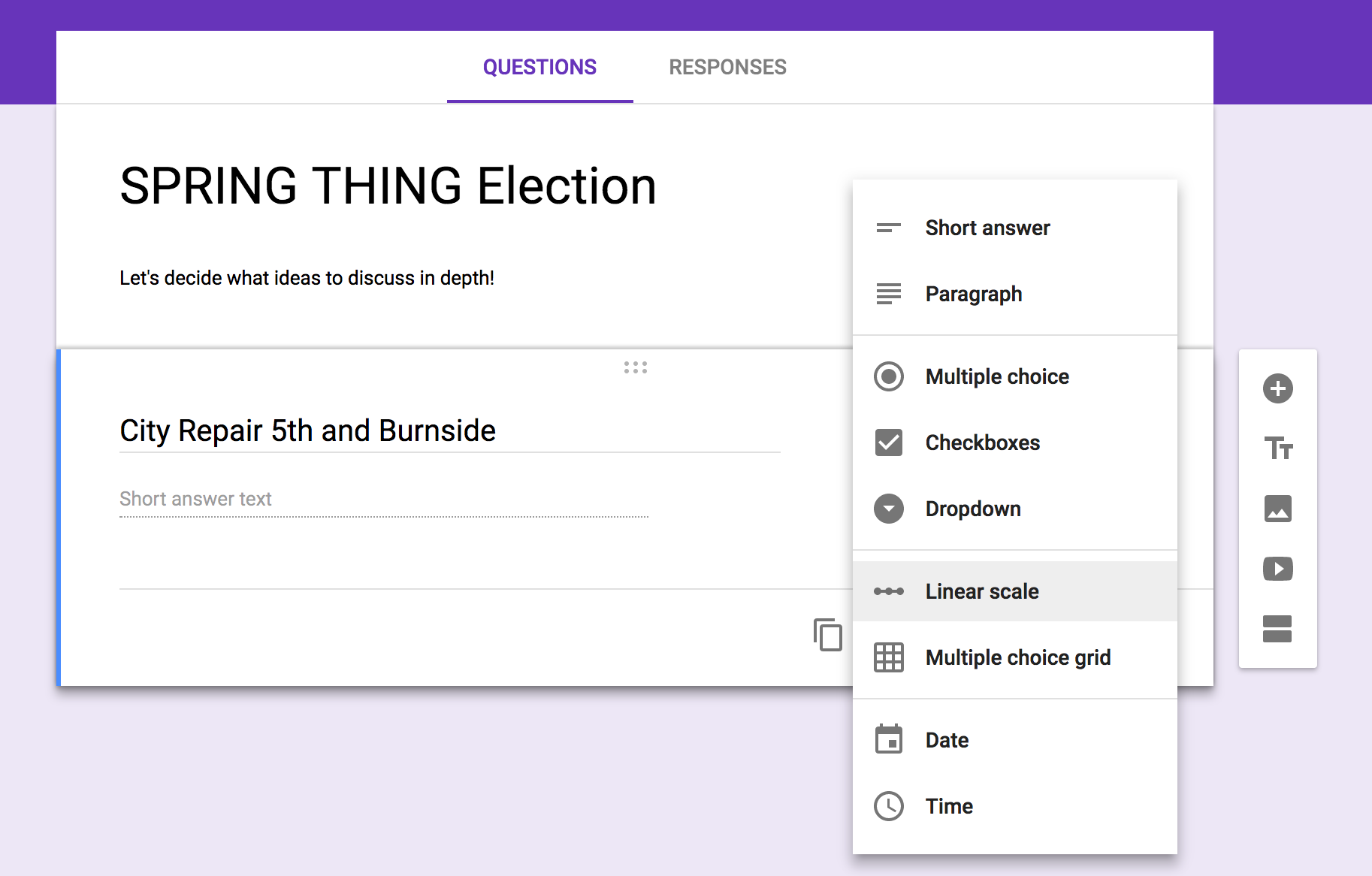Step 4: Set the scale start to 0

Each ballot entry should start at zero for the overall scale. Since Google Forms defaults to 1 as the starting value, you'll need to set it manually to 0.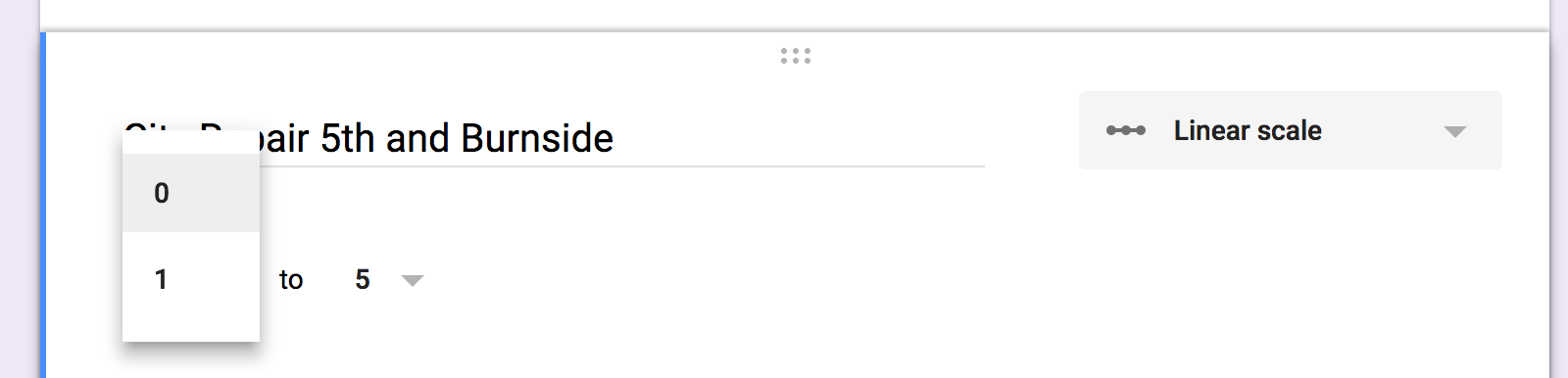Step 5: Make some more candidates on the ballot!

Might as well have at least three candidates on the ballot, since plurality voting does fine when there are only two options.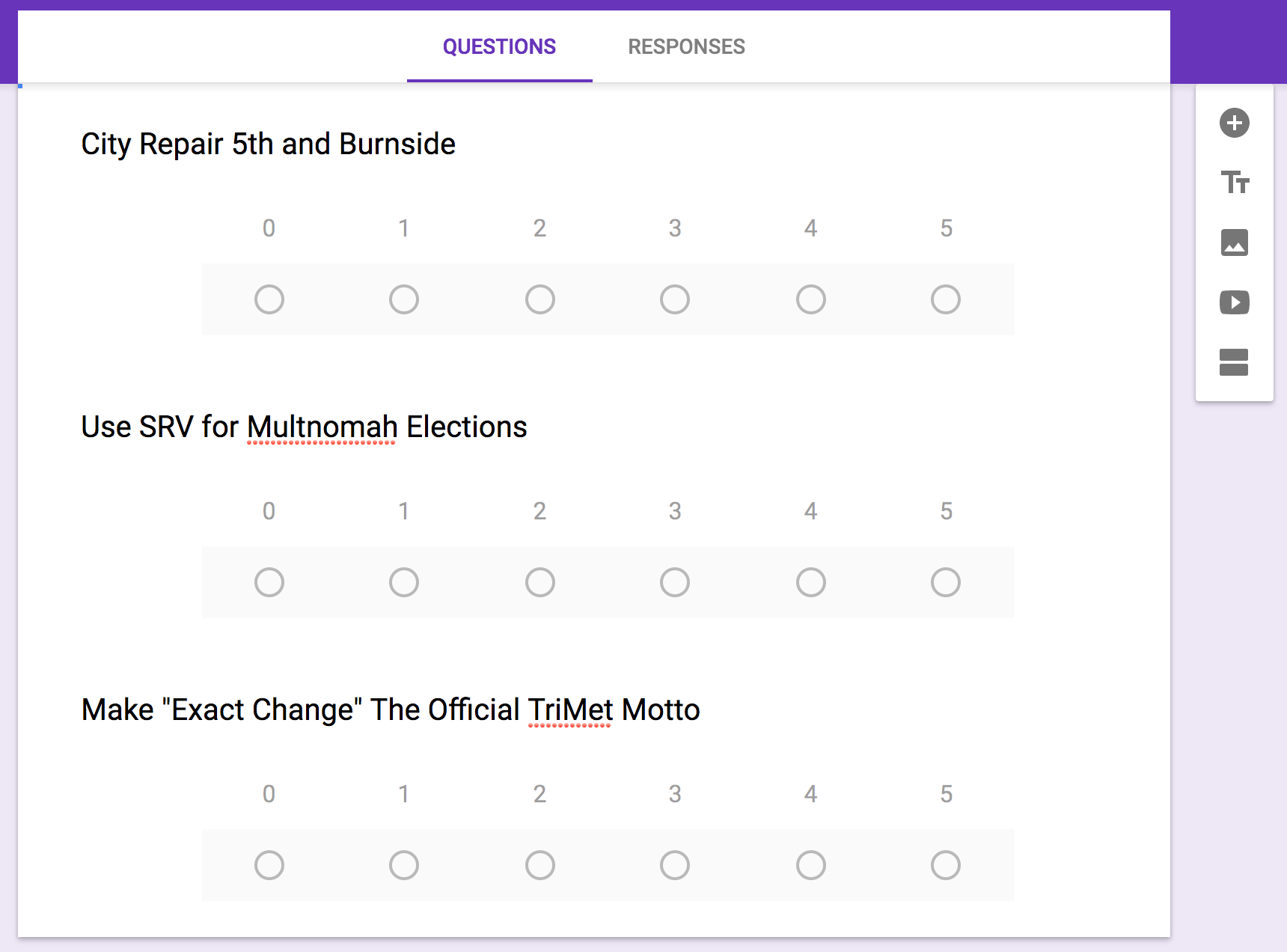Step 6: Open the Script Editor

From the action menu (the vertical "..." in the upper left), select the "< > Script Editor..." option. This is where you'll put the custom code to officiate the election from the form.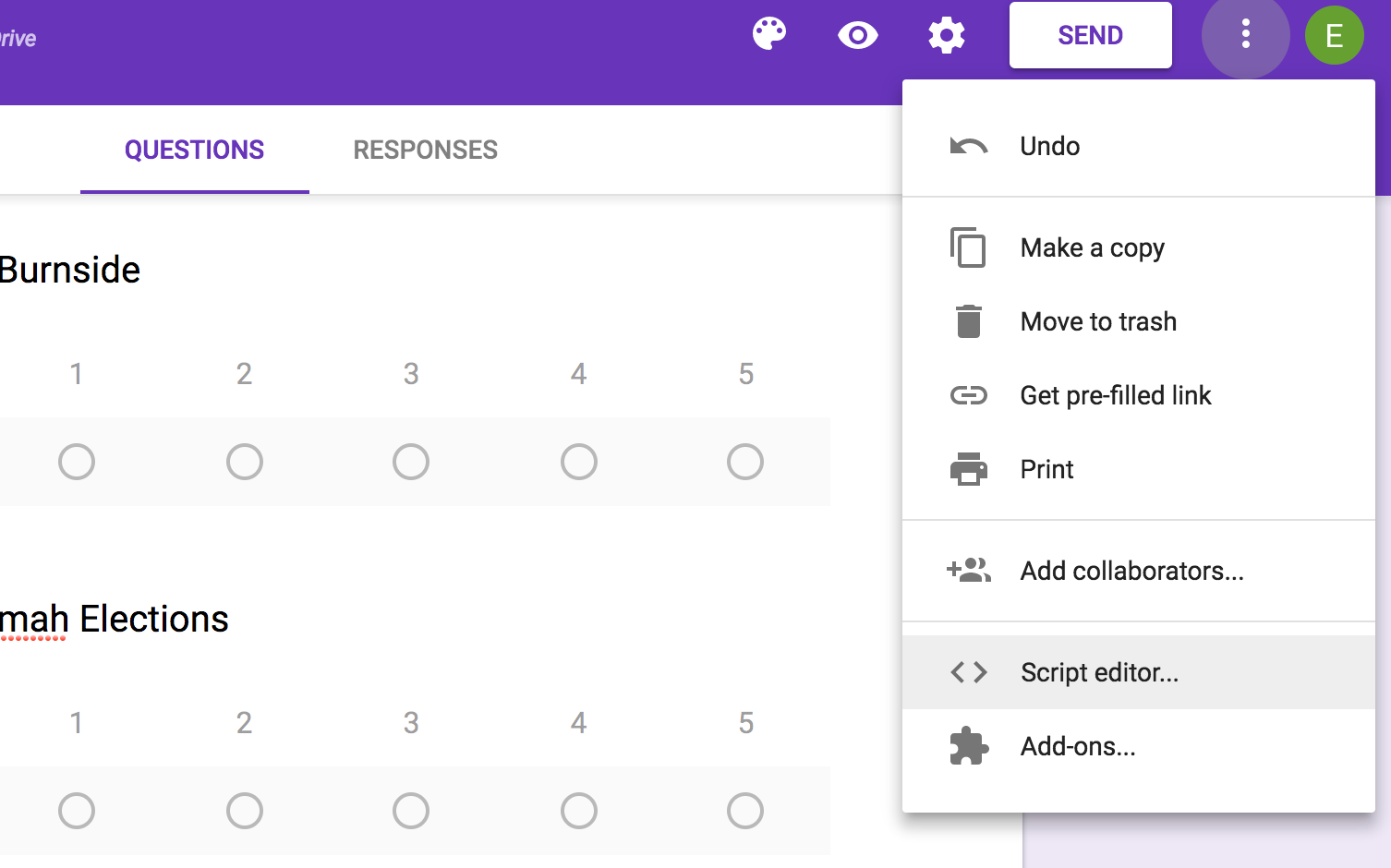Step 7: Replace all the code with the SRV calculator script

Copy the following code and paste it over the contents of the form's script.

``````
var ADDON_TITLE = 'SRV Calculator';

function onOpen(e) {
FormApp.getUi()
}

function onInstall(e) {
onOpen(e);
}

function runElection() {
var form = FormApp.getActiveForm();
var responses = form.getResponses();
if(responses.length == 0)
return;

candidate_names = [];
formItems = form.getItems();
for (var i = 0; i < formItems.length; i++)
candidate_names[i] = formItems[i].getTitle();

num_candidates = formItems.length;

var spreadsheet = SpreadsheetApp.create(form.getTitle() + " Results");

result_sheet.setName("Results");

result_range = result_sheet.getDataRange();
result_values = result_range.getValues();

num_ballots = responses.length;

// create empty score list and preference matrix
candidate_scores = [];
pref_matrix = [];
for(i = 0; i < num_candidates; i++)
{
candidate_scores[i] = 0;
pref_matrix[i] = [];
for(j = 0; j < num_candidates; j++)
pref_matrix[i][j] = 0;
}

for(b = 0; b < num_ballots; b++)
{
var ballot = responses[b].getItemResponses();
scores = [];
for(z = 0; z < num_candidates; z++)
scores[z] = 0;

for(i = 0; i < ballot.length; i++)
scores[ballot[i].getItem().getIndex()] = parseInt(ballot[i].getResponse());

for(c = 0; c < num_candidates; c++)
{
candidate_scores[c] += scores[c];
for(d = 0; d < num_candidates; d++)
if(scores[c] > scores[d])
pref_matrix[c][d] += 1;
}
}
function versus(x) { return 'vs. ' + x }
result_sheet.appendRow([ 'Election Results:' ]);
result_sheet.appendRow([ '', 'Total', 'Average Score'].concat(candidate_names.map( versus ) ) );
result_sheet.getRange(2, 2, 1, 2 + num_candidates).setHorizontalAlignment("right");

for(c = 0; c < num_candidates; c++)
{
pref_matrix[c][c] = '';
result_sheet.getRange(3 + c, 3).setNumberFormat("0.00");
result_sheet.getRange(3 + c, 4 + c).setBackgroundRGB(180, 180, 180);
}

// find the top two
top_a = 0;
top_b = 1;

for(c = top_b + 1; c < num_candidates; c++)
{
if(candidate_scores[c] > candidate_scores[top_a])
{
if(candidate_scores[top_a] > candidate_scores[top_b])
top_b = top_a;
top_a = c;
}
else if(candidate_scores[c] > candidate_scores[top_b])
{
top_b = c;
}
}
result_sheet.getRange(3 + top_a, 1, 1, 2).setBackgroundRGB(128, 255, 128);
result_sheet.getRange(3 + top_b, 1, 1, 2).setBackgroundRGB(128, 255, 128);
result_sheet.getRange(3 + top_a, 4 + top_b).setBackgroundRGB(128, 255, 128);
result_sheet.getRange(3 + top_b, 4 + top_a).setBackgroundRGB(128, 255, 128);

result_sheet.appendRow([' ']);
result_sheet.appendRow(['Top two are ' + candidate_names[top_a] + ", with a score of " + candidate_scores[top_a] + ", and " + candidate_names[top_b] + ", with a score of " + candidate_scores[top_b] + ", overall."]);
if(pref_matrix[top_a][top_b] >= pref_matrix[top_b][top_a])
{
winner = top_a;
loser = top_b;
}
else
{
winner = top_b;
loser = top_a;
}
result_sheet.appendRow([candidate_names[winner] + " was preferred over " + candidate_names[loser] + ", " + pref_matrix[winner][loser] + " to " + pref_matrix[loser][winner]+ "."] );
result_sheet.appendRow([' ']);
result_sheet.appendRow(['The winner is: ' + candidate_names[winner]]);
}
``````

When you're done it'll look like this: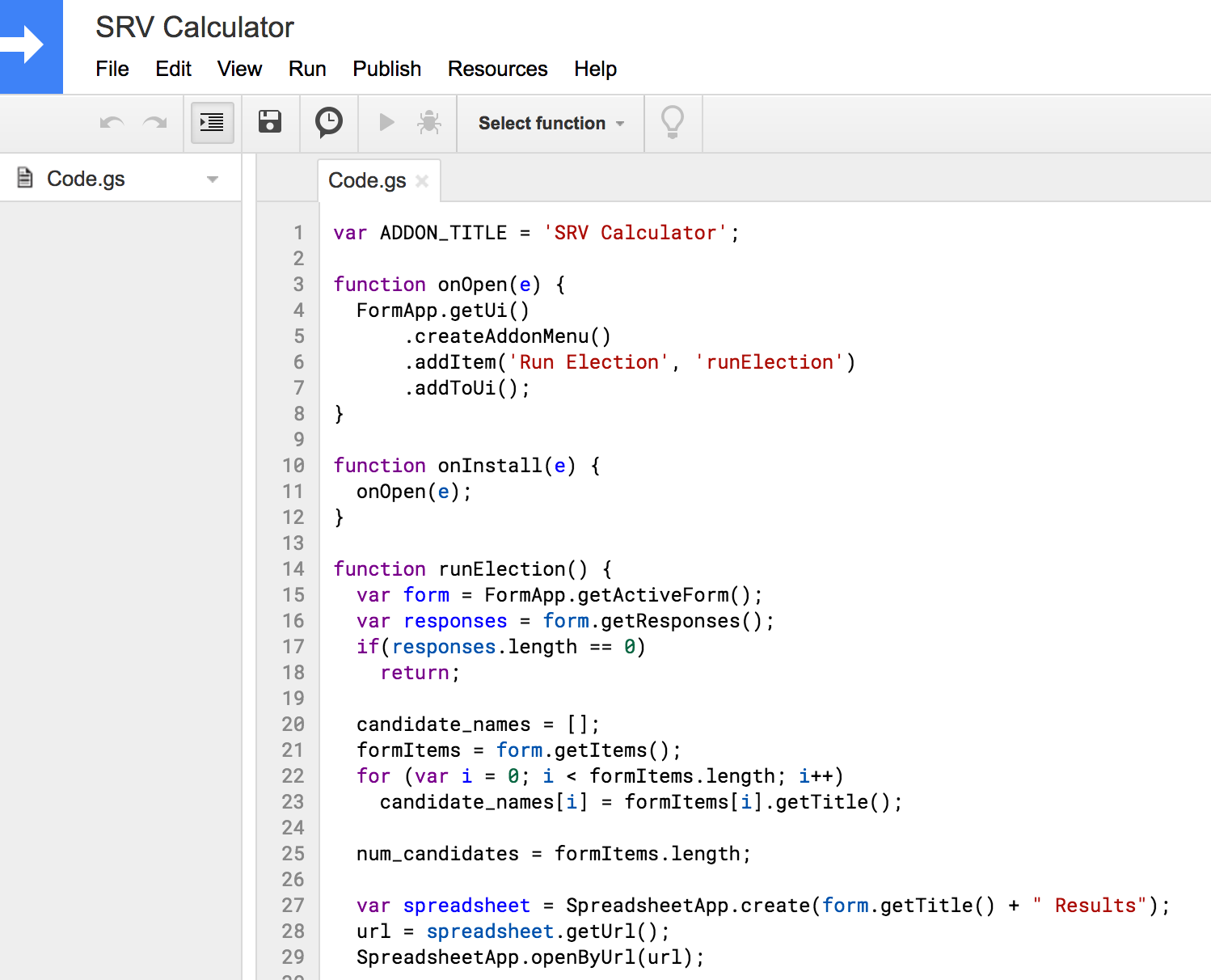Choose "Save" from the File menu. The script editor will ask you to save the script with a name. Call it "SRV Calculator:"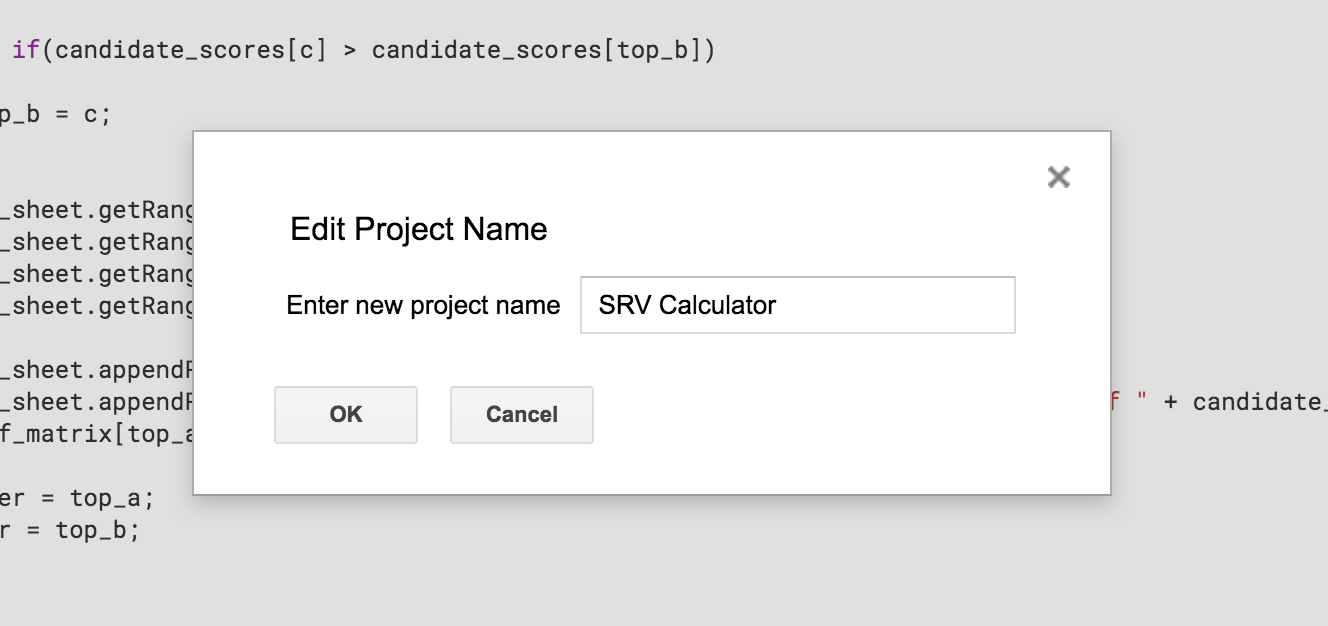Step 8: Send out the ballots!

You can send the ballots out in email form, or distribute a link to the ballot form via other means.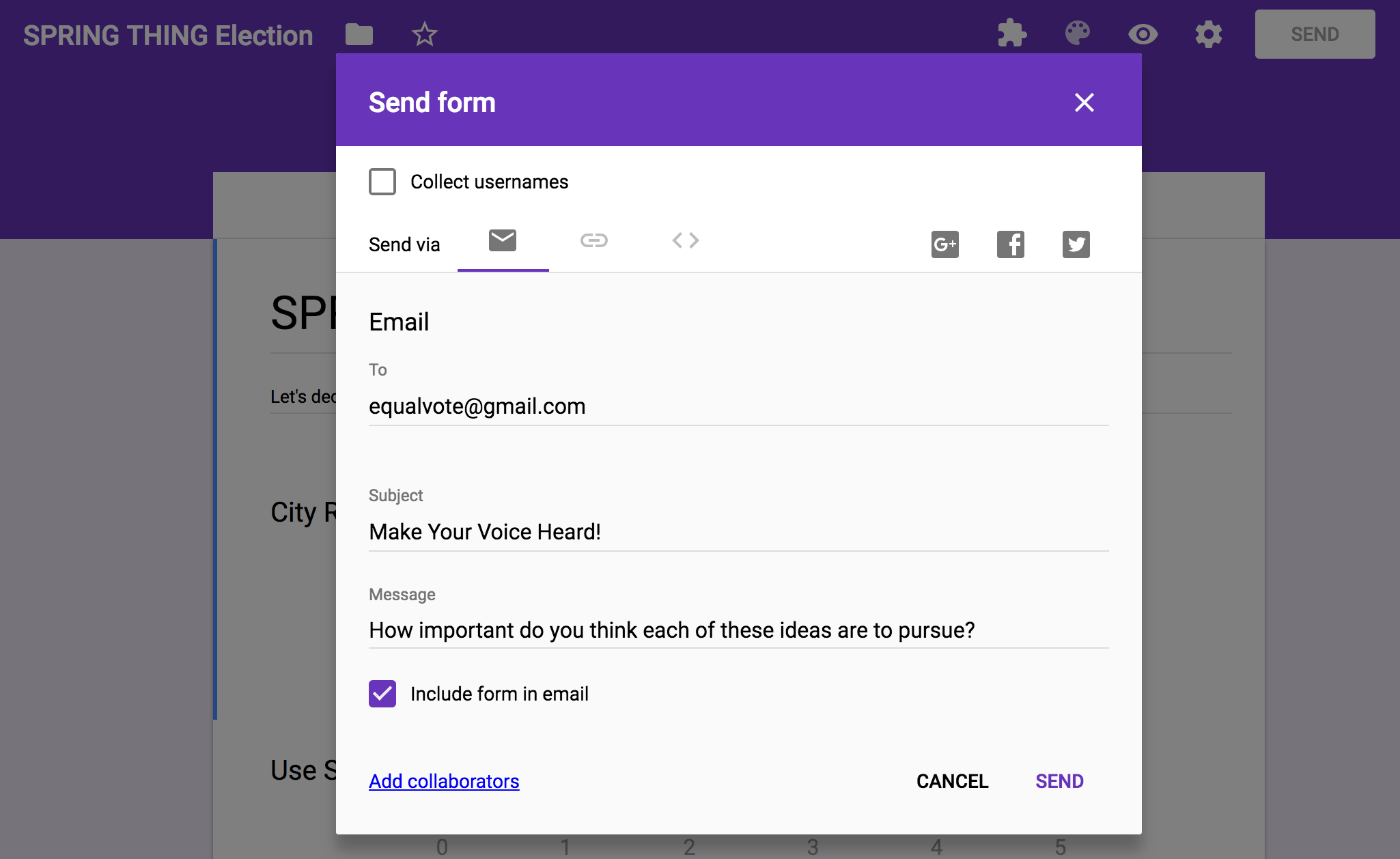If you checked the "Include form in email" option, users will be able to fill out the form within their email clients.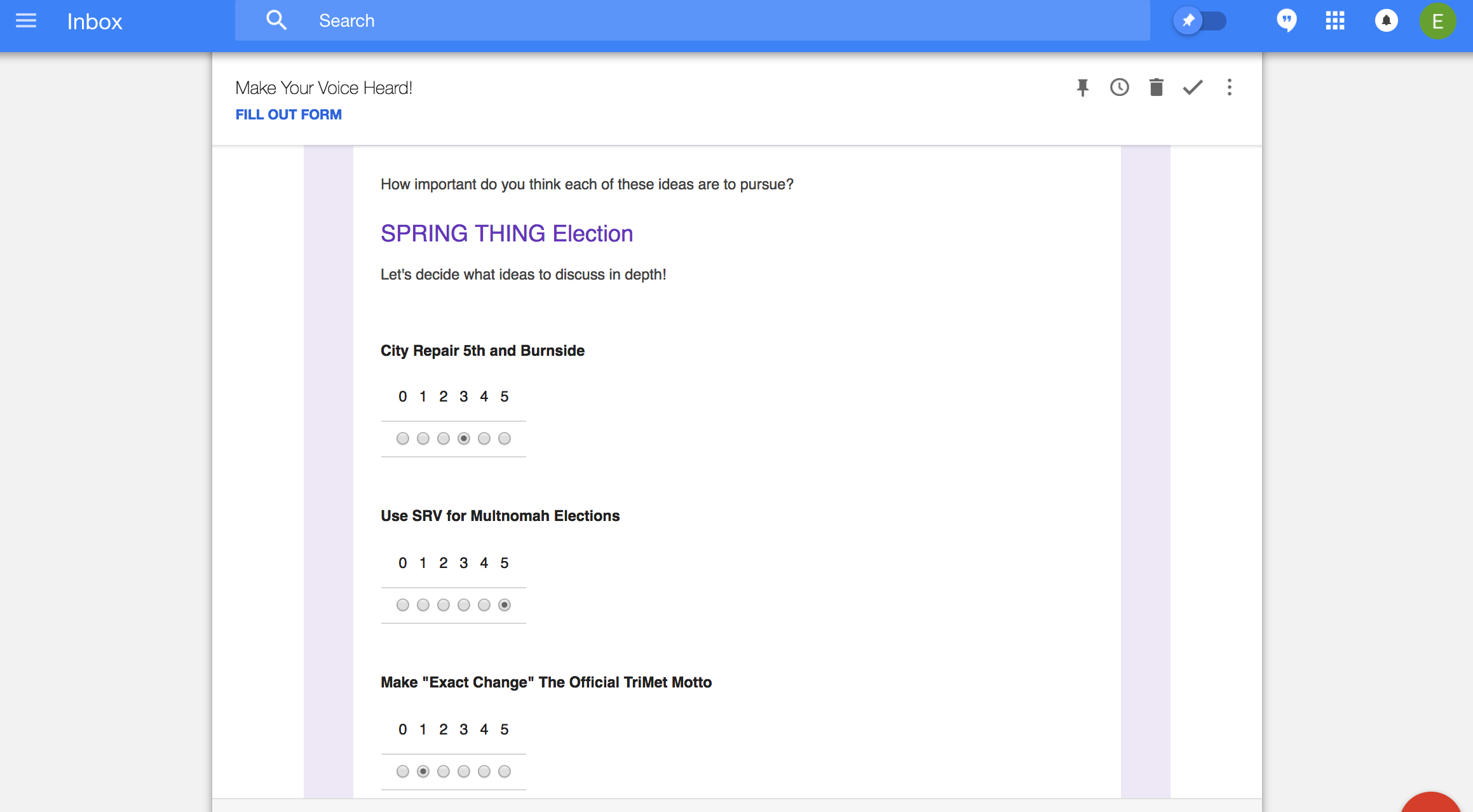Users can also fill out the form on the Google Forms site: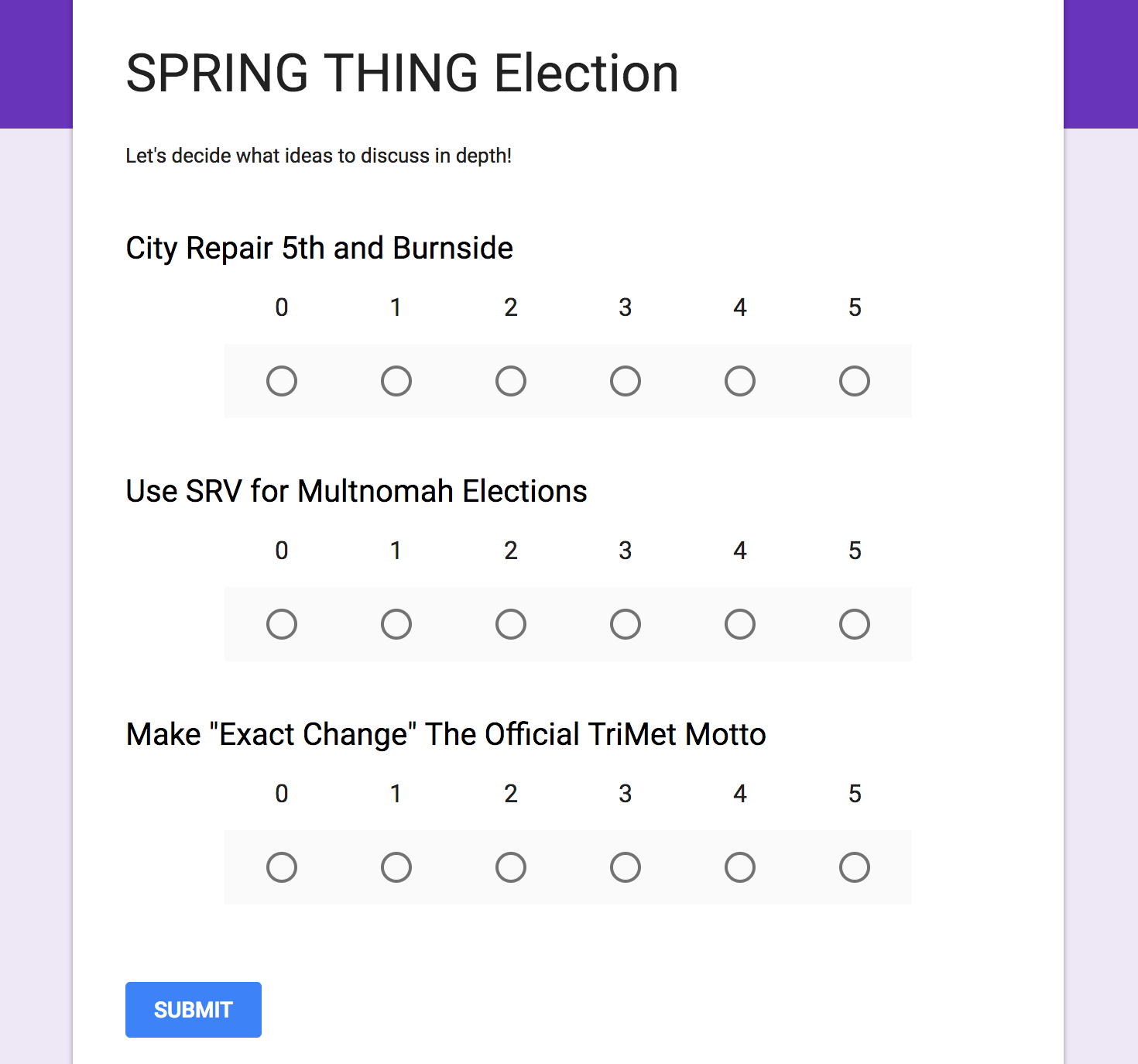Step 9: Run the election!

You'll need to close and re-open the form for the script option to show up in the Google Form. You can find the form easily in the "Recent" files section of your google drive: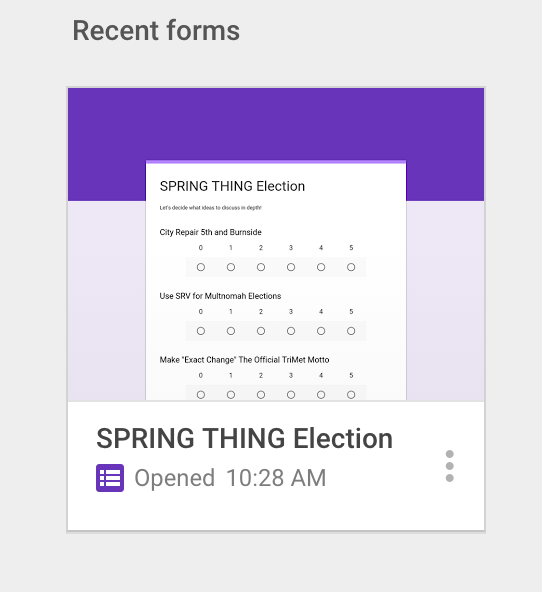Once you've re-opened the form, click the puzzle-piece add-ons icon and choose "SRV Calculator," and then choose "Run Election":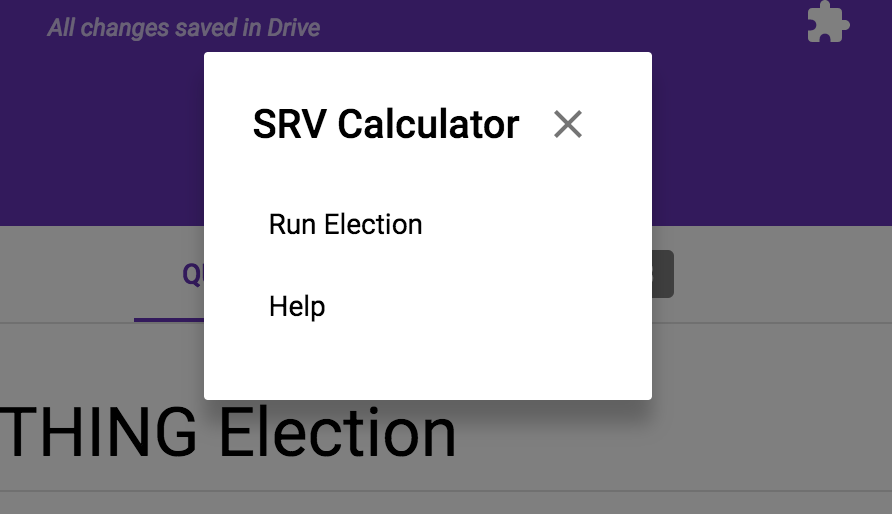Google will ask you to authorize the script to run on Google Forms and Google Spreadsheets. Go ahead and allow it: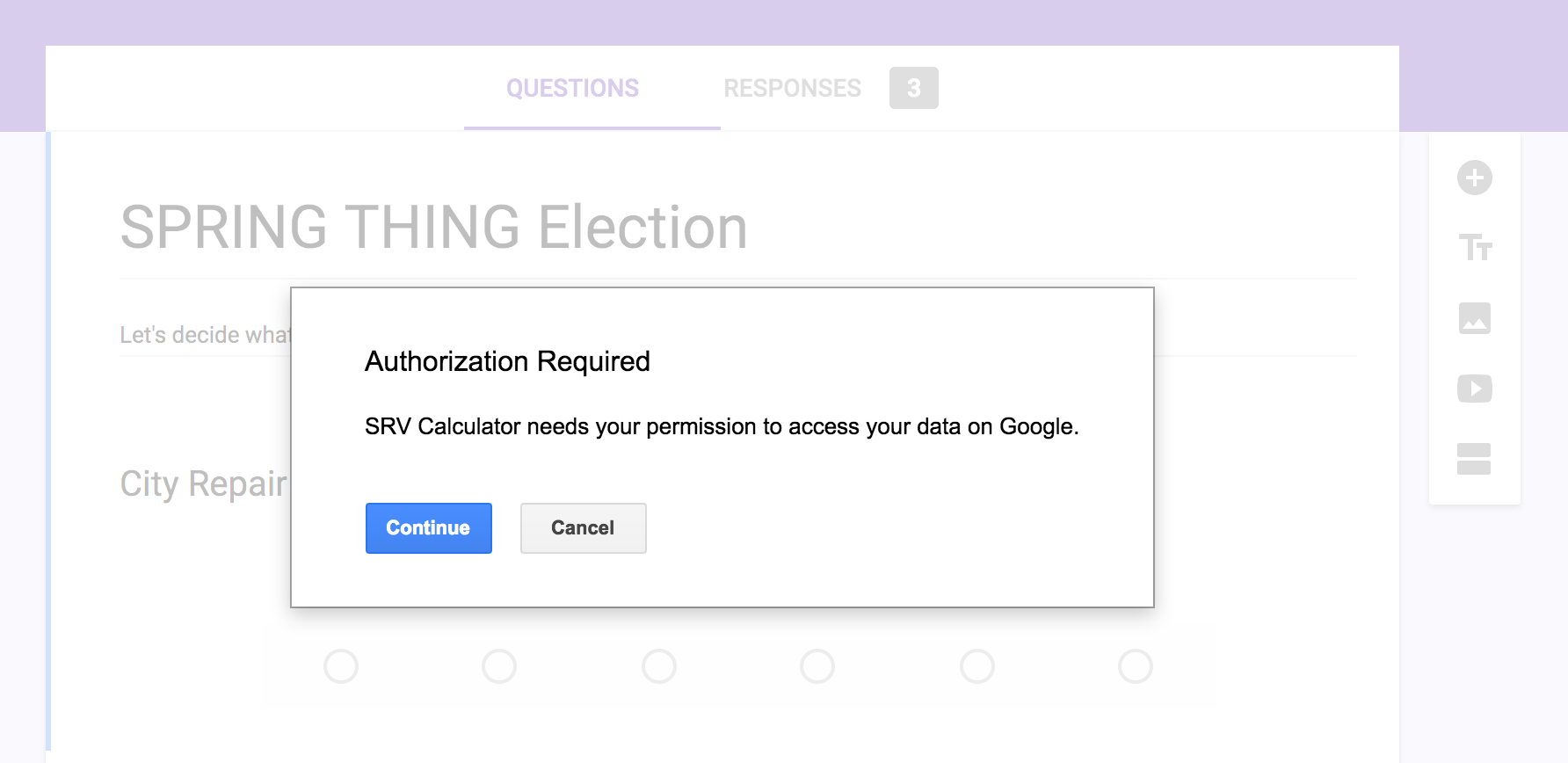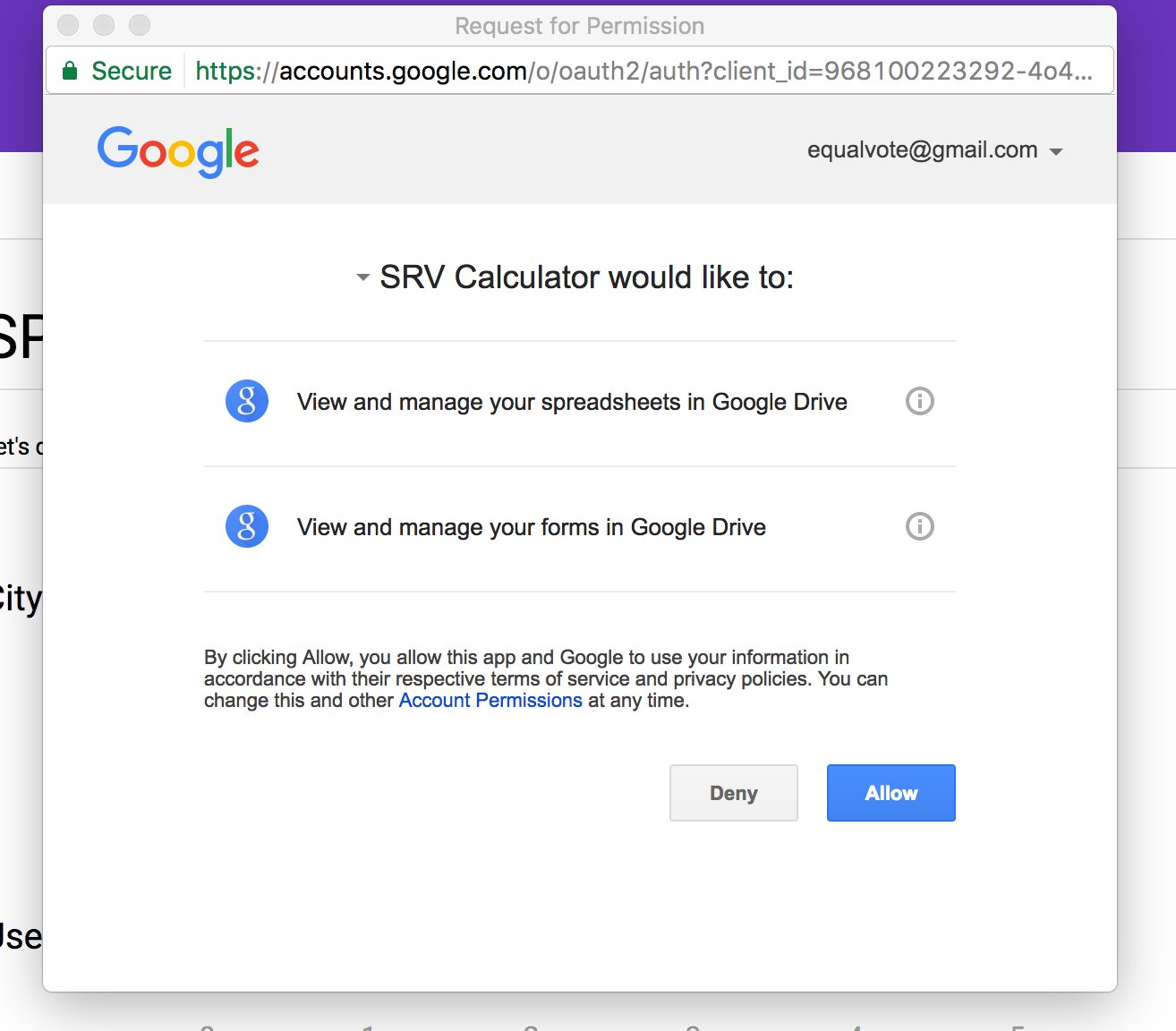Once the script has permission to run, a small dialog at the top of the page will show the progress. Once it's finished, the results page will be complete.

Step 10: Open the results

The SRV Calculator script creates a Google Sheet spreadsheet with the results of the election. You can find it most easily in the "Recent" files section in your Google Drive: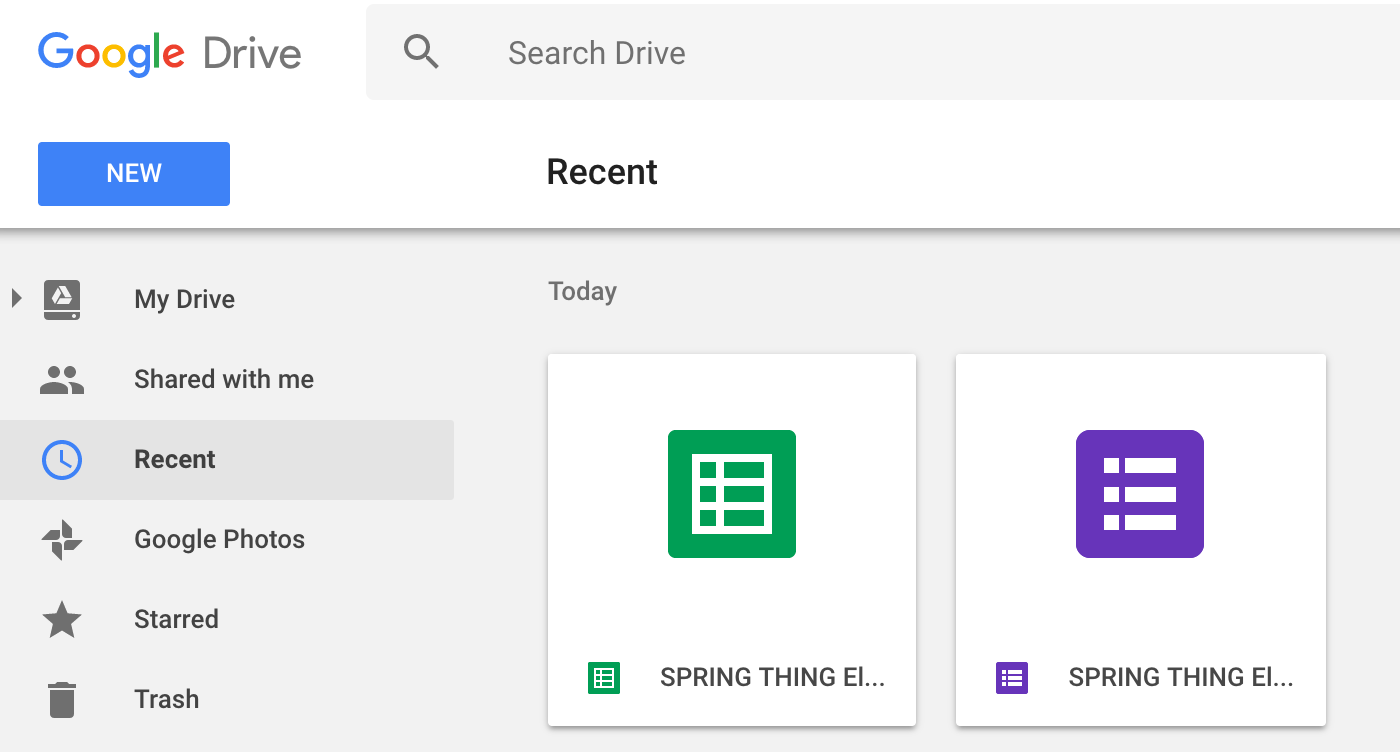Open the spreadsheet and see the results!